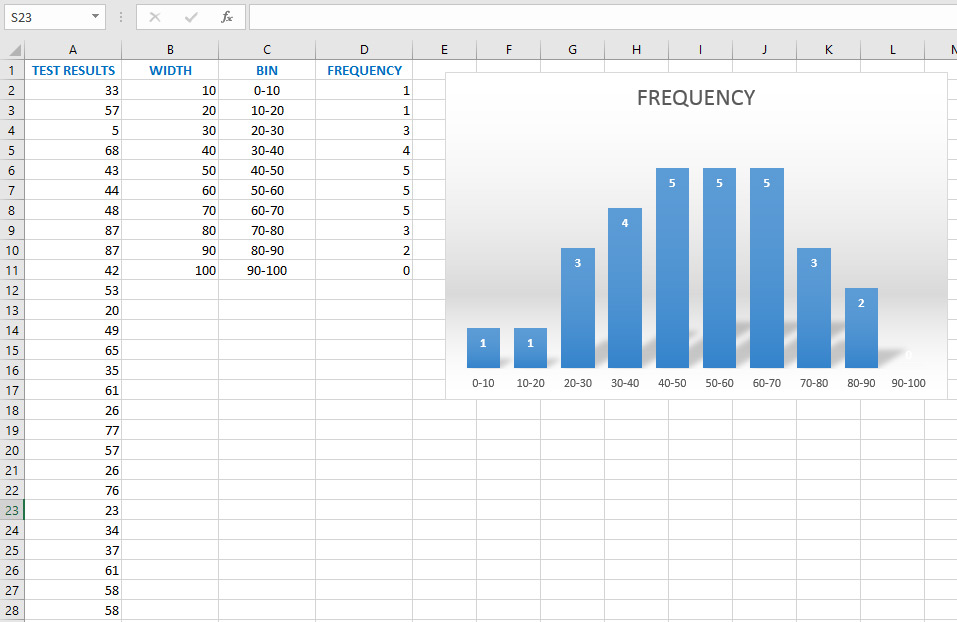# FREQUENCY function

The FREQUENCY function is used to calculate how often certain values ​​in a given range are repeated. This is an array function, and we can use it to calculate a distribution by which we can see how many points students have scored on a test and for other similar statistical analyzes. To help you understand how the function works will also illustrate this with a simple chart …

To begin with, a table with several columns is required. The first column (TEST RESULTS) contains the results of all tests, ie the number of points scored by each student. In the second column (WIDTH) we state the width of the bin. The number contained in it is the maximum value of the bin. We will also use it to form a third column (BIN) where we enter values ​​that we later use as labels in the chart. In the last column we will calculate the frequency.

It is necessary to select all the cells in which we want to calculate the frequency, and if we have values ​​coming out of the range we can select one cell more. Then we enter the formula:

=FREQUENCY (A2: A30; B2: B11)

The first argument to this function is the column in which the results are located, and the second argument is the one containing the bin widths. Since this is an array function, after entering the formula it is necessary to press CTRL + SHIFT + ENTER to finish. If done correctly the frequencies will be displayed within the column.

Next, let’s create a simple chart. Select the data in the FREQUENCY column and create a Column Chart. Format it as desired and select the data from the GROUP column for the x-axis labels. The result is a chart of frequency distribution.